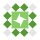Home Communities
IT Knowledge
Inspiration
Languages
EN

# C# / .NET - get current time in milliseconds

8 points
Created by:Veer-Ahmad
487

In this short article, we would like to show how to get current time in milliseconds in C# / .NET.

Motivation: the most common way in different systems to present time in milliseconds is UNIX timestamp that is measured as milliseconds elapsed from 1970-01-01 (e.g. JavaScript `Date` class that uses that time).

Quick solution:

``````DateTime unixEpoch = new DateTime(1970, 1, 1);

DateTime currentTime = DateTime.UtcNow;
TimeSpan elapsedTime = date.Subtract(currentTime);

long unixTimstamp = (long)elapsedTime.TotalMilliseconds;  // <--- unix timstamp in milliseconds``````

## 1. Custom Unix milliseconds timestamp example

In this section, we have prepared reusable code that returns Unix time.

``````public static class TimeUtils
{
public static long GetUnixTimstamp(DateTime date)
{
DateTime zero = new DateTime(1970, 1, 1);
TimeSpan span = date.Subtract(zero);

return (long)span.TotalMilliseconds;
}

public static long GetUnixTimstamp()
{
return GetUnixTimstamp(DateTime.UtcNow);
}
}``````

Example:

``Console.WriteLine(TimeUtils.GetUnixTimstamp());``

Output:

``1565647530865``

## 2. .NET 4.6 API Unix milliseconds timestamp example

.NET +4.6 introduced some API that lets to get Unix time in easy way.

``````DateTimeOffset now = (DateTimeOffset)DateTime.UtcNow;

Console.WriteLine(now.ToUnixTimeMilliseconds());``````

Output:

``1565647040281``

## 3. Custom milliseconds timestamp example

`Ticks` are measured from 12:00:00 midnight, January 1, 0001 in the Gregorian Calendar.

``````long time = DateTime.Now.Ticks / TimeSpan.TicksPerMillisecond;

Console.WriteLine(time);``````

Output:

``63701247264339``
Note: be careful using this example, because time here is not measured from 1970-01-01.

## References:

Join to our subscribers to be up to date with content, news and offers.